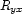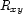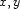#### `dom` method

This method translates a dominance literal `dom(X R Y)` into the corresponding problem-specific constraint (as described in Section 4.3.2). Remember that sinceis the inverse of, we only represent one of them: we representwhenare encoded by `I,J` and `I>J`.

`meth dom(X R Y)   {self var2node(X _)}   {self var2node(Y _)}   I = @var2int.X   J = @var2int.Yin    if I==J then 1::{Encode R}   elseif I>J then       @choices.(I*1000+J)::{Encode R}   else       @choices.(J*1000+I)::{Encode {Inverse R}}   end end`

Here is how to inverse and encode the symbolic representation of a dominance specification.

<<
`fun {Encode R}   case R   of eq    then 1   [] above then 2   [] below then 3   [] side  then 4   [] _|_   then {Map R Encode}   end end fun {Inverse R}   case R   of eq    then eq   [] above then below   [] below then above   [] side  then side   [] _|_   then {Map R Inverse}   end end`

Denys Duchier
Version 1.2.0 (20010221)